# NCERT Exemplar Problems and their Solutions

Reviewed By:
Krishna Kant Majee
M.Sc., B.Ed.

### Chapter 4-Carbon and Its Compounds-Short Answers

Q.30. Draw the electron dot structure of ethyne and also draw its structural formula.
Ethyne is a hydrocarbon with molecular formula $C_{2}H_{2}$. Here two carbon atoms are bonded together by a triple covalent bond and one hydrogen atom is bonded with each carbon atom through a single covalent bond. Hence the electron dot structure of ethyne molecule can be representes as: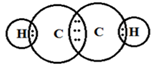Structural formula of ethyne is: H ─ C ≡ C ─ H
Q.31. Write the names of the following compounds.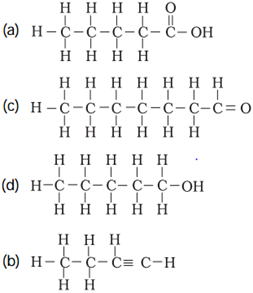(a) Pentanoic acid as it contains five carbon atoms with one –COOH group.
(b) Pentyne as it contains five carbon atoms in a straight chain with one triple covalent bond at terminal.
(c) Heptanal as it contains seven carbon atoms with one –CHO group ( aldehyde group)
(d) Pentanol because it contains 5 carbon atoms with one –OH group.

Q.32. Identify and name the functional groups present in the following compounds.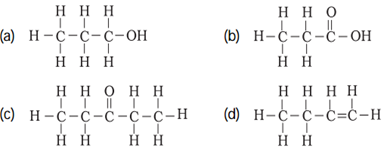(a) Functional group: ─ OH
Name of compound: Alcohol
(b) Functional group: ─ COOH
Name of compound: Carboxylic acid
(a) Functional group: >C = O
Name of compound: Ketone
(a) Functional group: ─ C = C ─
Name of compound: Alkene
Q.33. A compound X is formed by the reaction of a carboxylic acid $C_{2}H_{4}O_{2}$ and an alcohol in the presence of a few drops of $H_{2}SO_{4}$. The alcohol on oxidation with alkaline $KMnO_{4}$ followed by acidification gives the same carboxylic acid as used in this reaction. Give the names and structures of
(a) Carboxylic acid,
(b) Alcohol and
(c) The compound x. Also write the reaction.
(a) Carboxylic acid with molecular formula $C_{2}H_{4}O_{2}$ is acetic acid or ethanoic acid having the structure as: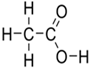(b) Given alcohol forms acetic acid on oxidation with alkaline $KMnO_{4}$ followed by acidification. Therefore, it must be ethanol with structure $CH_{3} ─ CH_{2} ─ OH.$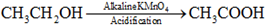(c) Reaction of ethanoic acid with ethanol in presence of a few drops of conc. $H_{2}SO_{4}$ is an esterification reaction that forms an ester, ethyl ethanoate $(CH_{3}COOC_{2}H_{5}).$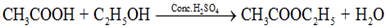Q.34. Why detergents are better cleansing agents than soaps? Explain.
Detergents and soaps both are cleansing agents but detergents can be used even in hard water whereas soaps cannot use in hard water.
Soaps forms an insoluble precipitate with calcium and magnesium ions found in hard water. This precipitate is called as scum which sticks to the cloths and makes the cleaning even more difficult.
On the contrary, detergents have charged ends and do not form insoluble precipitates with calcium and magnesium ions.
The cleansing action of detergents is stronger than soaps. They are more soluble in water therefore it is convenient to use them compare to soaps.
Q.35.Name the functional groups present in the following compounds.
(a) $CH_{3}COCH_{2}CH_{2}CH_{2}CH_{3}$
(b) $CH_{3}CH_{2}CH_{2}COOH$
(c) $CH_{3}CH_{2}CH_{2}CH_{2}CHO$
(d) $CH_{3}CH_{2}OH$
(a) $CH_{3}COCH_{2}CH_{2}CH_{2}CH_{3}$ – Ketone ( >C=O)
(b) $CH_{3}CH_{2}CH_{2}COOH$ – Carboxylic acid (─ COOH)
(c) $CH_{3}CH_{2}CH_{2}CH_{2}CHO$ – Aldehyde (─ CHO)
(d) $CH_{3}CH_{2}OH$ – Alcohol (─ OH)
Q.36. How is ethene prepared from ethanol? Give the reaction involved in it.
This conversion is possible through dehydration reaction which occurs in the presence of concentrated sulphuric acid or concentrated phosphoric (V) acid, $H_{3}PO_{4}$ at 160°C.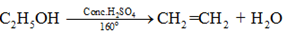Q.37. Intake of small quantity of methanol can be lethal. Comment
When methanol is entered in our body, it is metabolized in the liver and form formaldehyde which further converted to formate. Formate is essential for survival because it acts as building block for many biomolecules but high levels of it can cause toxicity.
Formaldehyde also reacts with living cells and can cause coagulation of protoplasm. Excess of methanol in our body also affects the optic nerve and can cause blindness.
Q.38. A gas is evolved when ethanol reacts with sodium. Name the gas evolved and also write the balanced chemical equation of the reaction involved.
When ethanol reacts with sodium metal, it forms sodium ethoxide $(C_{2}H_{5}O^{-}Na^{+})$ and hydrogen gas is released. The reaction is as follows.
$2C_{2}H_{5}OH + 2Na \rightarrow 2C_{2}H_{2}O^{-}Na^{+} + H_{2}\uparrow$
So the gas is hydrogen gas that is evolved during the reaction.
Q.39. Ethene is formed when ethanol at 443 K is heated with excess of concentrated sulphuric acid. What is the role of sulphuric acid in this reaction? Write the balanced chemical equation of this reaction.
Answer. Conversion of ethanol to ethene is a dehydration reaction which occurs in the presence of excess of concentrated sulphuric acid. Here concentrated sulphuric acid acts as dehydrating agent and remove water molecule from ethanol to form ethene.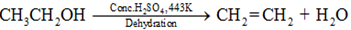Hence concentrated sulphuric acid acts as a dehydrating agent as it removes water molecule.
Q.40. Carbon, group (14) element in the periodic table, is known to form compounds with many elements.
Write an example of a compound formed with
(a) Chlorine (group 17 of periodic table)
(b) Oxygen (group 16 of periodic table)
(a) Carbon (C) exhibits tetravalnecy and form four covalent bonds with other elements. Chlorine atom contains 7 valence electrons and forms one covalent bond with other elements. The compound that is formed by combination of carbon and chlorine is carbon tetrachloride.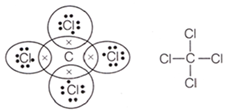(b) Oxygen can form two covalent bonds and form carbon dioxide with carbon atom. The center carbon atom is bonded with two oxygen atoms through double covalent bonds. Hence the structure of carbon dioxide would be O=C=O.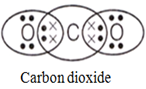Q.41. In electron dot structure, the valence shell electrons are represented by crosses or dots.
(a) The atomic number of chlorine is 17. Write its electronic configuration.
(b) Draw the electron dot structure of chlorine molecule.
(a) Atomic number of chlorine atom is 17. Hence the electronic configuration of Cl (17)would be;
K L M
2 8 7
(b) Chlorine molecule is formed by formation of single covalent bond between two chlorine atoms. The electron dot structure of chlorine molecule is: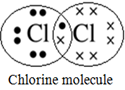Q.42. Catenation is the ability of an atom to form bonds with other atoms of the same element. It is exhibited by both carbon and silicon. Compare the ability of catenation of the two elements. Give reasons.
Both carbon (C) and silicon (Si) are placed in 14th group of the periodic table and have four valence electrons in their valence shell.
Both of them can form four covalent bonds with other elements and can also exhibit the phenomenon of catenation.
Catanation is the self linkage property of atoms due to which they can bond with other similar atoms to form long chain compounds. It depends on the bond energy of atoms.
Out of carbon and silicon, carbon exhibits catenation much more than silicon. This is because of smaller size of carbon and high bond energy of C-C bonds compare to Si-Si bonds. Hence carbon shows catenation to a greater extent than silicon.
Q.43. Unsaturated hydrocarbons contain multiple bonds between the two c-atoms and show addition reaction. Give the test to distinguish ethane from ethene.
Answer. Ehene is an unsaturated hydrocarbon as it contains a double covalent bond between two carbon atoms.
Presence of un-saturation or double covalent bond can be distinguished with the help of bromine solution in $CCl_{4}$. It is an orange colour solution which shows colour change after addition in ethene solution.
The orange colour disappears because of formation of the colourless ethylene dibromide
$(CH_{2}Br ─ CH_{2}Br).$ It is an addition reaction of ethene due to presence of double covalent bond in the molecule.
$CH_{2} = CH_{2} + Br_{2}\rightarrow CH_{2}Br ─ CH_{2}Br$
Ethane is a saturated hydrocarbon and cannot show this test. It burns with a clear flame whereas unsaturated hydrocarbons burn with yellow flame and lots of black smoke.
Q.44. Match the reactions given in Column I with the names given in Column II.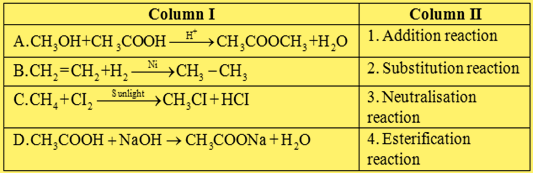Answer. The matching of Column I and Column II is as given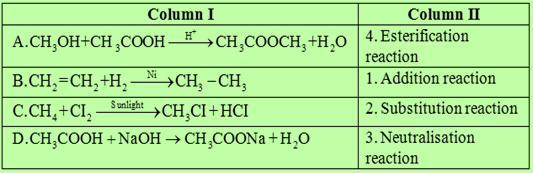Q.45. Write the structural formulae of all the isomers of hexane.
Hexane can exhibit five isomers with molecular formula $C_{6}H_{14}$.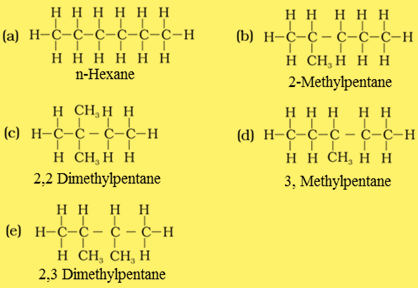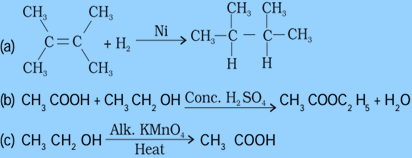(c) This reaction involves the conversion of alcohol (ethanol) to carboxylic acid (acetic acid). It is an oxidation reaction and alkaline $KMnO_{4}$ acts as an oxidising agent for ethanol.